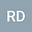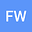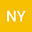Existence of positive solutions of second-order delayed differential system on infinite interval
•••• +1
• Ran Ding,
• Fanglei Wang,
• Nannan Yang,
• Yuanfang RU
Ran Ding
Hohai University
Author ProfileFanglei Wang
College of Science, Hohai University
Author ProfileNannan Yang
Hohai University
Author ProfileYuanfang RU
China Pharmaceutical University
Author Profile## Abstract

The present paper is focused on the analysis on the existence of positive solutions of a second order differential system with two delays

\begin{equation} \left\{\begin{array}{lcr}x_{1}^{\prime\prime}(t)-a_{1}(t)x_{1}(t)+m_{1}(t)f_{1}(t,x(t),x_{\tau}(t))=0,\ \ t>0,\\ x_{2}^{\prime\prime}(t)-a_{2}(t)x_{2}(t)+m_{2}(t)f_{2}(t,x(t),x_{\tau}(t))=0,\ \ t>0,\\ x_{1}(t)=0,\ \ -\tau_{1}\ \leq t\leq 0,\;\text{and}\;\lim_{t\rightarrow\infty}x_{1}(t)=0,\\ x_{2}(t)=0,\ \ -\tau_{2}\ \leq t\leq 0,\;\text{and}\;\lim_{t\rightarrow\infty}x_{2}(t)=0\end{array}\right.\nonumber \\ \end{equation}

by using two well-known fixed point theorems.

#### Peer review status:UNDER REVIEW

23 Feb 2020Submitted to Mathematical Methods in the Applied Sciences
28 Feb 2020Assigned to Editor
28 Feb 2020Submission Checks Completed
10 Jul 2020Reviewer(s) Assigned